# Samacheer Kalvi 6th Science Solutions Term 2 Chapter 2 Electricity

You can Download Samacheer Kalvi 6th Science Book Solutions Guide Pdf, Tamilnadu State Board help you to revise the complete Syllabus and score more marks in your examinations.

## Tamilnadu Samacheer Kalvi 6th Science Solutions Term 2 Chapter 2 Electricity

### Samacheer Kalvi 6th Science Electricity Textual Evaluation

I. Choose the appropriate answer.

Samacheer Kalvi 6th Science Guide Term 2 Question 1.
The device which converts chemical energy into electrical energy is
(a) fan
(b) solar cell
(c) cell
(d) television
(c) cell

Science Term 2 Question 2.
Electricity is produced in
(a) transformer
(b) power station
(c) electric wire
(d) television
(b) power station

Samacheer Kalvi 6th Science Term 2 Question 3.
Choose the symbol for battery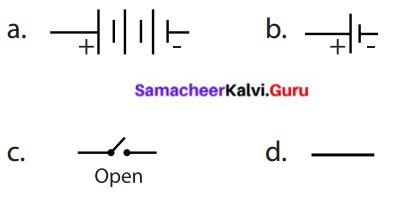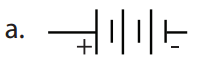Samacheer Kalvi Guru 6th Term 2 Question 4.
In which among the following circuits does the bulb glow?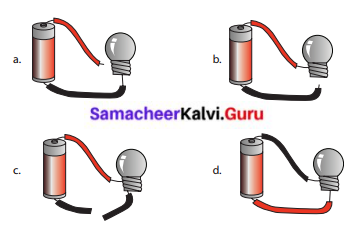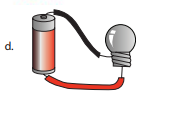Samacheer Kalvi Guru 6th Science Question 5.
……… is a good conductor
(a) silver
(b) wood
(c) rubber
(d) plastic
(a) silver

II. Fill in the blanks.

1. _______ are the materials which allow electric current to pass through them.
2. Flow of electricity through a closed circuit is _______
3. _______ is the device used to close or open an electric circuit.
4. The long perpendicular line in the electrical symbol represents its _______ terminal.
5. The combination of two or more cells is called a _______

1. conductors
2. a complete electric circuit
3. Key or Switch
4. positive
5. Battery

III. True or False. If False, give the correct statement.

Term 2 Science Book Question 1.
In a parallel circuit, the electricity has more than one path.
True

Samacheer Kalvi Term 2 Question 2.
To make a battery of two cells, the negative terminal of one cell is connected to the negative terminal of the other cell.
False. To make a battery of two cells, the negative terminal of one cell is connected to the positive terminal of the other cell. (OR) To make a battery of two cells, the positive terminal of one cell is connected to the negative terminal of the other cells.

Kalvi Guru 6th Science Question 3.
The switch is used to close or open an electric circuit.
True

Samacheer Kalvi Guru 6th Standard Question 4.
Pure water is a good conductor of electricity.
False. Impure water is a good conductor of electricity

Samacheer Guru 6th Science Question 5.
Secondary cell can be used only once.
False. Primary cells can be used only once

IV. Match the following :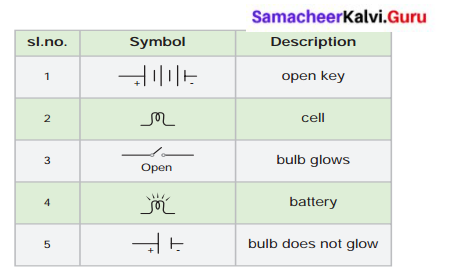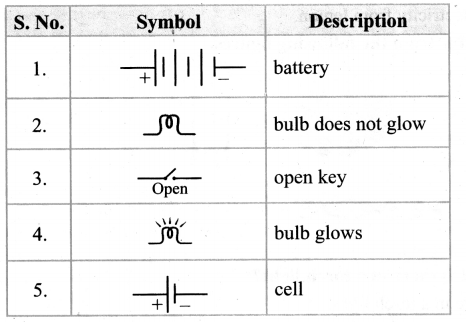V. Arrange in sequence: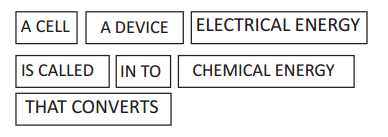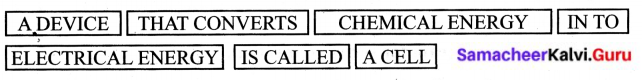VI. Give Very Short Answers:

Samacheer Kalvi Guru 6th Solutions Question 1.
In the given circuit diagram, which of the given switch(s) should be closed, So that only the bulb A glows.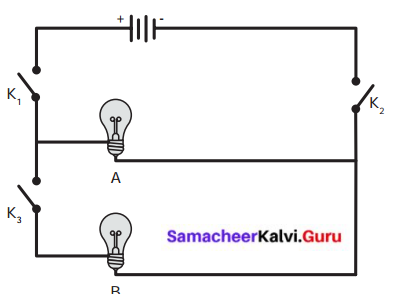Switches K1 and K2 should be closed.

Question 2.
Assertion (A): It is very easy for our body to receive electric shock.
Reason (R) : Human body is a good conductor of electricity.
a. Both A and R are correct and R is the correct explanation for A.
b. A is correct, but R is not the correct explanation for A.
c. A is wrong but R is correct.
d. Both A and R are correct and R is not the correct explanation for A.
a. Both A and R are correct and R is the correct explanation for A.

Question 3.
Can you produce electricity from lemon?
Yes, I can produce electricity from lemon.

Question 4.
Identify the conductor from the following figures.The conductor is Iron chain.

Question 5.
What type of circuit is there in a torchlight?
Simple circuit system is used in a torchlight.

Question 6.
Circle the odd one out. Give reason for your choice.
Switch, Bulb, Battery, Generator.
Generator. Switch, Bulb, Battery are the components used to make simple circuit. Generator is used to generate electricity.

VII. Give Short Answers:

Question 1.
Draw the circuit diagram for series connection.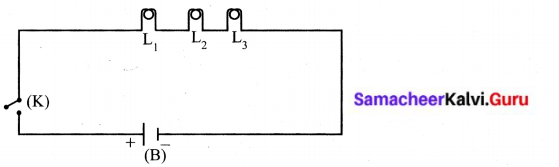Question 2.
Can the cell used in the clock gives us an electric shock? Justify your answer.

1. The cell used in clock will not give us an electric shock because the voltage of that cell is very low nearly 1.5 v.
2. So it will not affect our body.

Question 3.
Silver is a good conductor but it is not preferred for making electric wires. Why?
Silver is a good conductor. But it is a costly metal. So it is not preferred for making electric wire.

VIII. Answer in detail.

Question 1.
What is the source of electricity? Explain the various power stations in India? Thermal power stations:

1. In thermal power stations, thermal energy is generated by burning coal, diesel or gas to produce steam.
2. Steam is used to rotate turbine to generate electricity.
3. Here heat energy is converted into electric energy.

Hydel power stations :

1. In hydel power stations, the turbine is made to rotate by the flow of water from dams.
2. Here kinetic energy is converted into electric energy.

Atomic power station :

1. Here nuclear energy is used to boil water
2. The steam thus produced is used to rotate the turbine, as a result electricity is produced.
3. Here nuclear energy is converted into mechanical energy.

Windmills :

1. In wind mills, wind energy is used to rotate turbine to produce electricity.
2. Here kinetic energy is converted into electrical energy.

Question 2.
Tabulate the different components of an electric circuit and their respective symbols.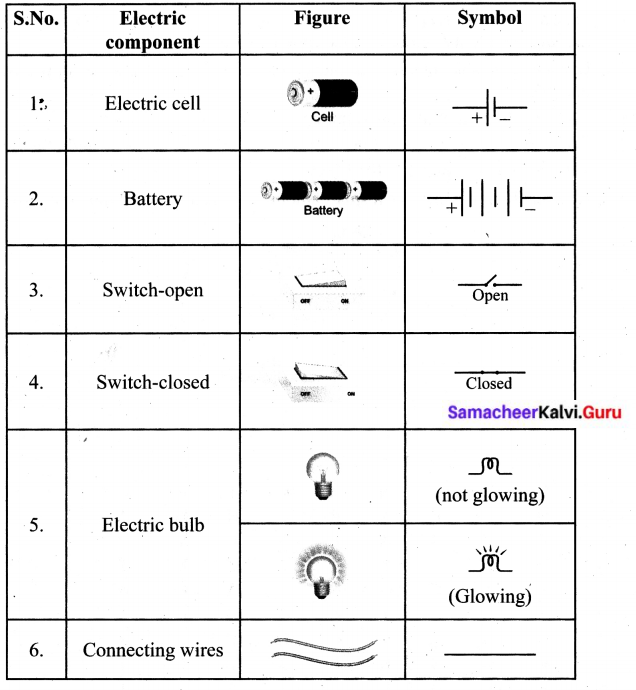Question 3.
Write short notes on conductors and insulators.
Conductors:
The rate of flow of electric charges in a circuit is called electric current. The materials which allow electric charges to pass through them are called conductors.
Examples: Copper, iron, aluminum, impure water, earth etc.,

Iniulaton (Non-Conductors):
The materials which do not allow electric charges to pass through them are called insulators or non- conductors. Examples: plastic, glass, wood, rubber, china clay, ebonite etc.

IX. Questions Based on Higher Order Thinking Skills.

Question 1.
Rahul wants to make an electric circuit. He has a bulb, two wires, a safety pin and a piece of copper. He does not have any electric cell or battery. Suddenly he gets some idea. He uses a lemon instead of a battery and makes a circuit. Will the bulb glow?
Yes the bulb will glow:

1. Take a lemon. Squeeze it without breaking its skin. The squeezing action releases the juice inside the lemon needed as a the battery to work.
2. Use a nail to make one hole in one end of the lemon and push a copper wire into that hole.
3. Then, push the nail into the other end.
4. Connect a bulb with one of the terminal with the copper wire and other terminal with the nail, as shown in the figure.
5. Now the lemon generates a small amount of electricity and the bulb glows.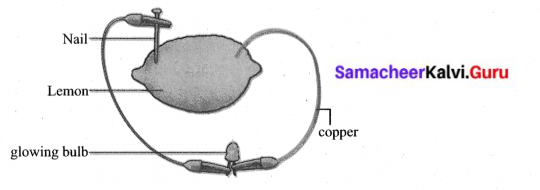X. Search ten words in the given word grid and classify them as conductors and insulators.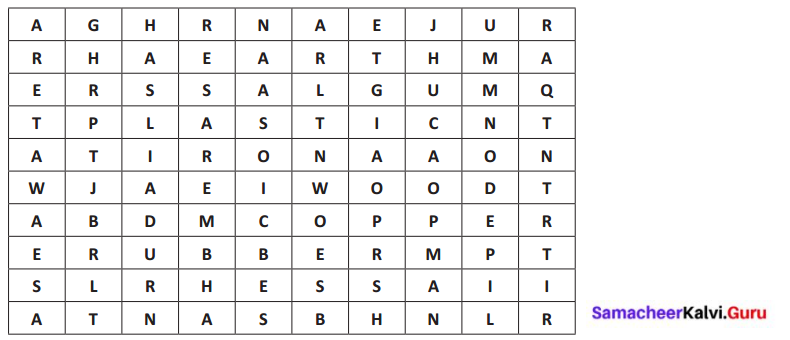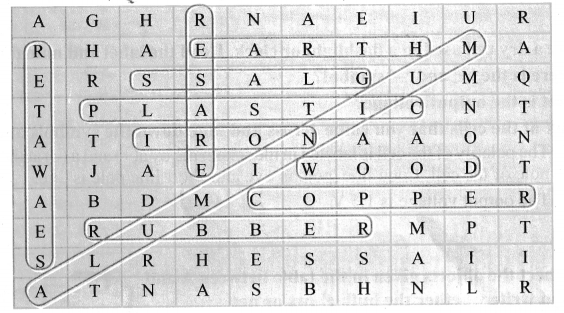Conductors:

1. Alumtnium
2. Earth
3. Copper
4. Iron
5. Sea water

Insulators:

1. Wood
2. Plastic
3. Rubber
4. Glass
5. Eraser

### Samacheer Kalvi 6th Science Electricity Intext Activities

Activity 1

Question 1.
List out the electrical appliances used in your home.

1. Television
2. Computer
3. Laptop
4. Mobile Phones
5. Fridge
6. Heater
7. Air conditioner
8. Microwave oven
9. Inverter
10. Washing machine with drier.

Activity 2

Question 2.
From the following pictures, identify those use primary cell and secondary cell. Mark Primary cell as ‘P’, Secondary cell as ‘S’.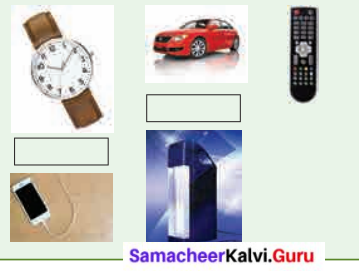Watch – Primary cell
Car – Secondary cell
Remote – Primary cell
Mobile phone – Secondary cell
Emergency Lamp – Secondary cell

Activity 3

Question 1.
Take a dry cell used in a flashlight or clock. Read the label and note the following.
1. Where is the ‘+’ and ‘-’ symbol?
2. What is the output voltage?
Look at the cells that you come across and note down the symbols and voltage.
1. The polarity of the cell is labeled in the schematic by a (+) and a (-) at the appropriate pole of the cell. Long line pole is +ve and short line pole is -ve.
2. The output voltage is 1.5 V.

Activity 4

Question 1.
Connect the objects given in the table between A and B and write whether the bulb glows or not.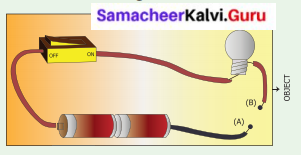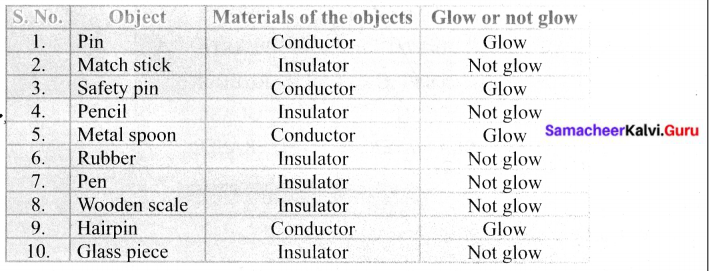Activity 5

Question 1.
Shall we make our own Battery
Produce electricity using copper plates, zinc plate, connecting wires, key, beaker and porridge (rice water) [the older the porridge the better will be the current| Arrange copper and zinc plates in series as shown in the figure. Half till two beakers with porridge. Connect the copper plate with the positive of and LED bulb and zinc to the negative. Observe what happens.
Now you can replace porridge with curd, potato, lemon etc.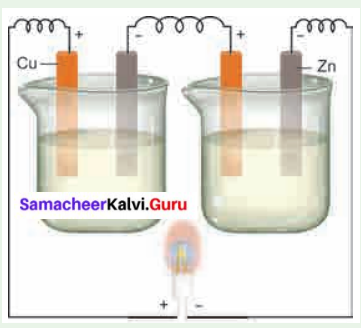Porridge, curd, potato, lemon are used to produce electricity. Less amount of electricity is produced. The LED bulb will glow with low energy.

### Samacheer Kalvi 6th Science Electricity Additional Questions

I. Choose the correct answer:

Question 1.
One of the atomic power station is located in ________
(a) Mettur
(b) Papanasam
(c) Neyveli
(d) Kalpakkam
(d) Kalpakkam

Question 2.
Coil of wire is rotated between electromagnets is created
(a) Heat
(b) Electromagnetic induction
(c) Kinetic energy
(d) None
(b) Electromagnetic induction

Question 3.
Secondary cells are used in ________
(a) Mobile phone
(b) wall clocks
(c) watches
(d) toys
(a) Mobile phone

Question 4.
Which circuits are used in homes?
(a) Parallel circuit
(b) Serial circuit
(c) Simple circuit
(d) None
(a) Parallel circuit

Question 5.
________ is an instrument used in electric circuits to find the quantity of current flowing through the circuit.
(a) Volt meter
(b) Ammeter
(c) Cell
(d) Key
(b) Aniniele

Question 6.
Thomas Alva Edison invented more than ________ useful inventions which are used in homes.
(a) 100
(b) 10
(c) 500
(d) 1000
(d) 1000

II. Fill in the blanks.

1. Hydel electricity is produced in ________ in Tirunelveli district.
2. In Thermal power station ________ is used as fuel.
3. ________ Cells used in automobiles like cars and buses are large and very heavy.
4. The rate of flow of electric charges in a circuit is called ________
5. ________ is a kind of fish which is able to produce electric current.
6. ________ are used to connect devices.
7. ________ was an American inventor, who invented electric bulb.

1. PaPanasam
2. Coal or diesel
3. Secondary
4. Electric Current
5. Electric Eel
6. Connecting Wires
7. Thomas Alva Edison

III. Find whether the following sentences are true or false. If false Correct the statement.

Question 1.
Windmills are located at Neyveli in Kanyakumari district.
False. Windmills are located at Aalvaimozhi in Kanyakumari district.

Question 2.
In atomic power station nuclear energy is converted into electrical energy.
True.

Question 3.
Primary cells are usually produced in large sizes.
False. Primary cells are usually produced in small sizes.

Question 4.
Primary cells are used in mobile phones.
False. Secondary cells are used in mobile phones.

Question 5.
In a circuit if the key is in open (off) condition, then electricity will not flow.
True.

Question 6.
Ebonite do not allow electric charges to pass through them.
True.

IV. Analogy.

Question 1.
Thermal power station : Neyveli.
Hydel power station : ________
Mettur

Question 2.
Kayatharu in Tirunelveli district: Wind mills.
Koodankulam in Tirunelveli district: ________
Atomic power station.

Question 3.
Primary cells : Toys
Secondary cells: ________
Emergency lamps.

Question 4.
Bulbs are connected in series : Series circuit.
Bulbs are connected in parallel: ________
Parallel circuit.

V. Match the following :
A.

 1. Thermal power stations (a) Kinetic energy converted into electrical energy 2. Hydel power stations (b) Nuclear energy is converted into mechanical energy and then electrical energy 3. Atomic power stations (c) Wind energy is used to produce electricity. 4. Windmills (d) Heat energy is converted into electrical energy.

1. – d
2. – a
3. – b
4. – c

B.

 i. Source of electricity (a) Conductors ii. To connect devices (b) Bulb iii. Consumes electricity (c) Insulators iv. Allow electric charges (d) Connecting wires v. Do not allow electric charges (e) Cell

1. – e
2. – d
3. – b
4. – a
5. – c

VI. Give Short Answers

Question 1.
How many cells are required to make a battery?

1. Two or more cells are combined to make a battery.
2. A battery is a collection of cells.

Question 2.
Where are the Hydel power stations located in Tamil Nadu?

1. Mettur in Salem District.
2. Papanasam in Tirunelveli District.

Question 3.
Where are Windmills located in Tamil Nadu?

1. Aralvaimozhi in Kanyakumari District.
2. Kayatharu in Tirunelveli District.

Question 4.
What are the types of electric circuits?

1. Simple circuit
2. Series circuit
3. Parallel circuit

Question 5.
What are the types of cells? Give examples.
(i) Primary cells.
(ii) Secondary cells.
Primary cells : Cells used in clocks, watches and toys, etc.
Secondary cell: Cells used in Mobile phones, Laptops and Emergency lamps.

Question 6.
What is the electric current?
The rate of flow of electric charges in a circuit is called electric current.

Question 7.
Differentiate Primary and Secondary cells.
Primary cells:

1. They cannot be recharged.
2. They can be used only once
3. Usually produced in small size.

Secondary cells:

1. They can be recharged.
2. They can be used again and again.
3. The size can be small or even large.

Question 8.
Define simple circuit.
A circuit consisting of a cell, key, bulb and connecting wires is called a simple circuit.

Question 9.
What is electric circuit?
An electric circuit is the continuous or unbroken closed path along which electric current flows from the positive terminal to the negative terminal of the battery.

Question 10.
Give the types of circuits.
They are three types of circuits.

1. Simple circuit
2. Series circuit
3. Parallel circuit.

Question 11.
Why parallel circuits are used in homes?
Parallel circuits are used in homes, because if any one of the bulb is damaged or disconnected, the other part of the circuit will work.

Question 12.
Identify the conductors and insulators among the following?
Copper, plastic, glass, iron, wood, Aluminum, China clay, impure water, Earth, ebonite
Conductors:

1. Copper
2. iron
3. Aluminium
4. impure water
5. Earth

Insulators:

1. plastic
2. glass
3. wood
4. China clay
5. ebonite

Question 13.
Draw a circuit diagram for parallel connection?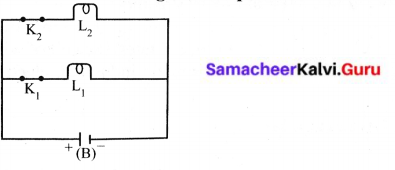VII. Answer in detail.

Question 1.
Describe open circuit and closed circuits with diagram.
(i) Open Circuit:
In a circuit if the key is in open (off) condition, then electricity will not flow and the circuit is called an open circuit. The bulb will not glow in this circuit.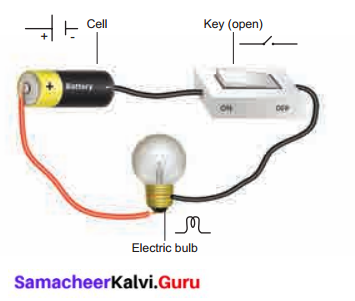(ii) Closed Circuit
In a circuit if the key is in closed (on) condition, then electricity will flow and the circuit is called a closed circuit. The bulb will glow in this circuit.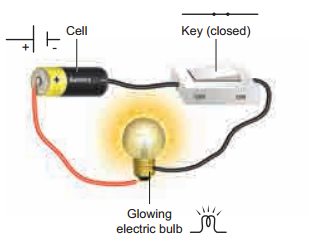Question 2.
Describe series circuit a..d parallel circuit with diagram.
(i) Series Circuit: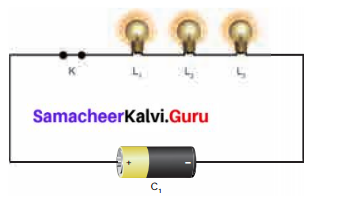If two or more bulbs are connected in series in a circuit, then that type of circuit is called series circuit. if any one of the bulbs is damaged or disconnected, the entire circuit will not work.

(ii) Parallel Circuit: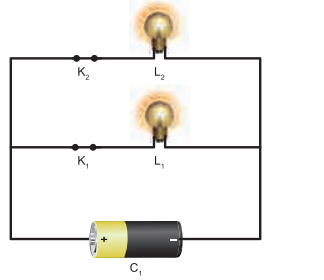If two or more bulbs are connected in parallel in a circuit, then that type of circuit is called parallel circuit. If any one of the bulb ¡s damaged or disconnected, the other part of the circuit will work. So parallel circuits are used in homes.

Question 3.
What are the safety measures to safeguard a person from electric shock?